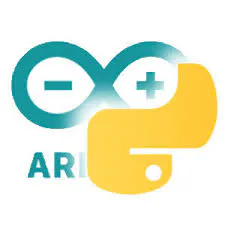# Autonomous Nerf Sentry Turret

Use computer vision to control a Nerf gun, aim, and fire, all on its own!

## Things used in this project

### Hardware components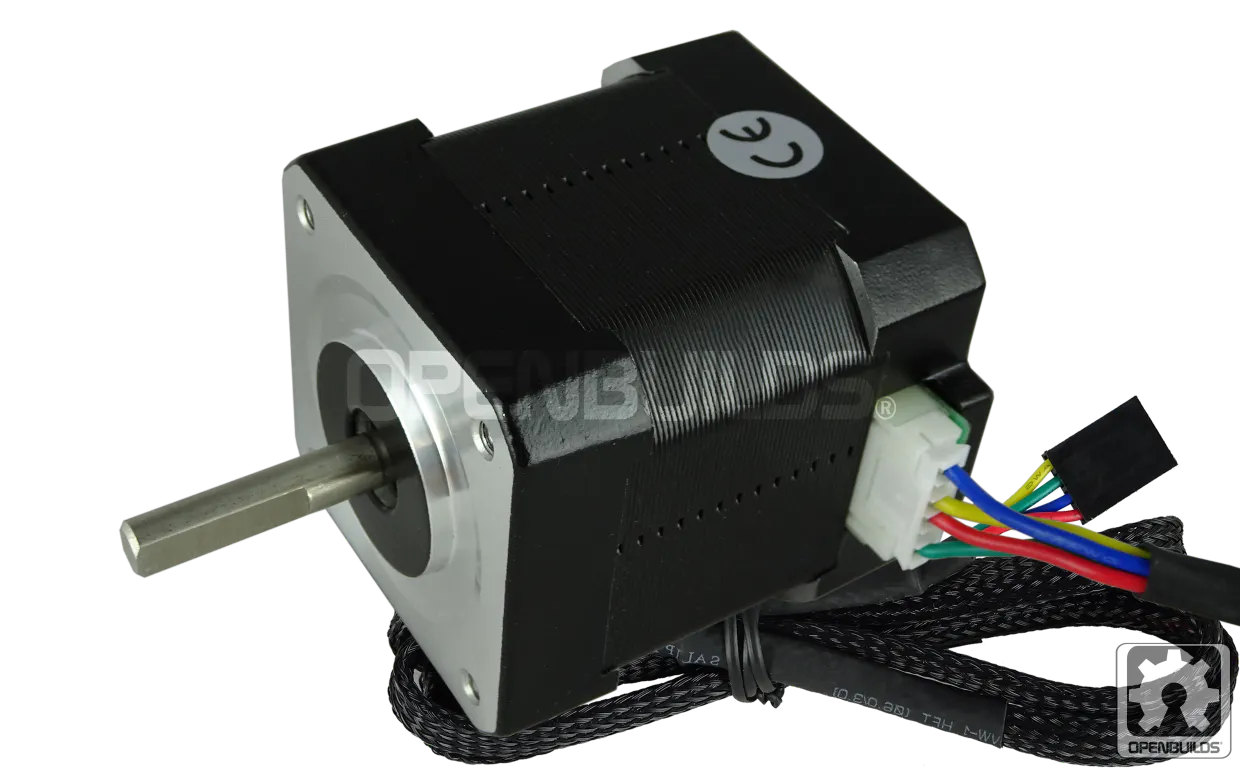OpenBuilds NEMA 17 Stepper Motor
×1
 DFRobot Stepper Motor with Gearbox
×1
 DFRobot Stepper Motor Driver
×1
 DFRobot Pixy 2 Cam
×1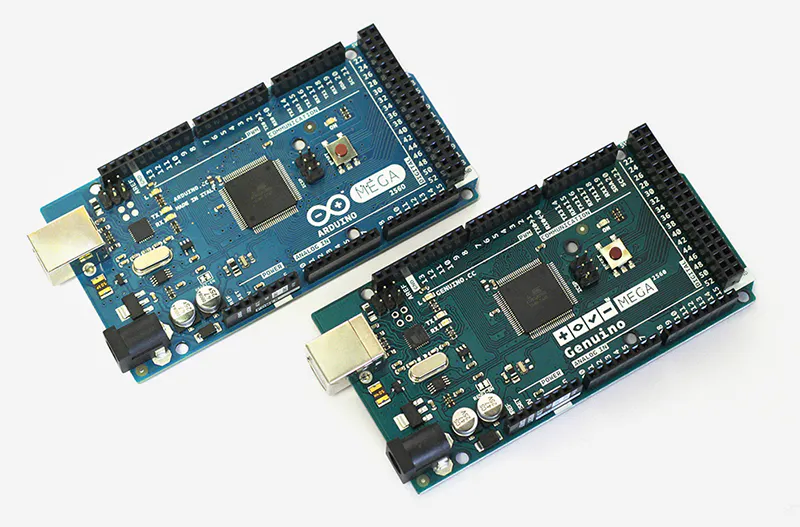Arduino Mega 2560 & Genuino Mega 2560
×1Ultrasonic Sensor - HC-SR04 (Generic)
×1
 Nerf Nitron Gun
×1

### Software apps and online servicesArduino IDE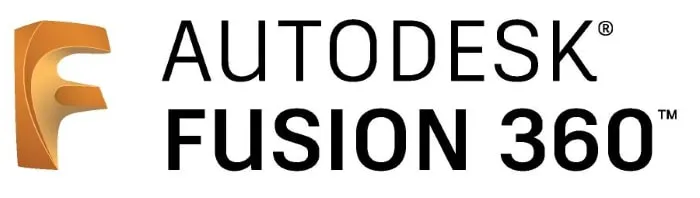Autodesk Fusion 360

### Hand tools and fabrication machines3D Printer (generic)Soldering iron (generic)
 CNC Router

## Schematics

### Schematic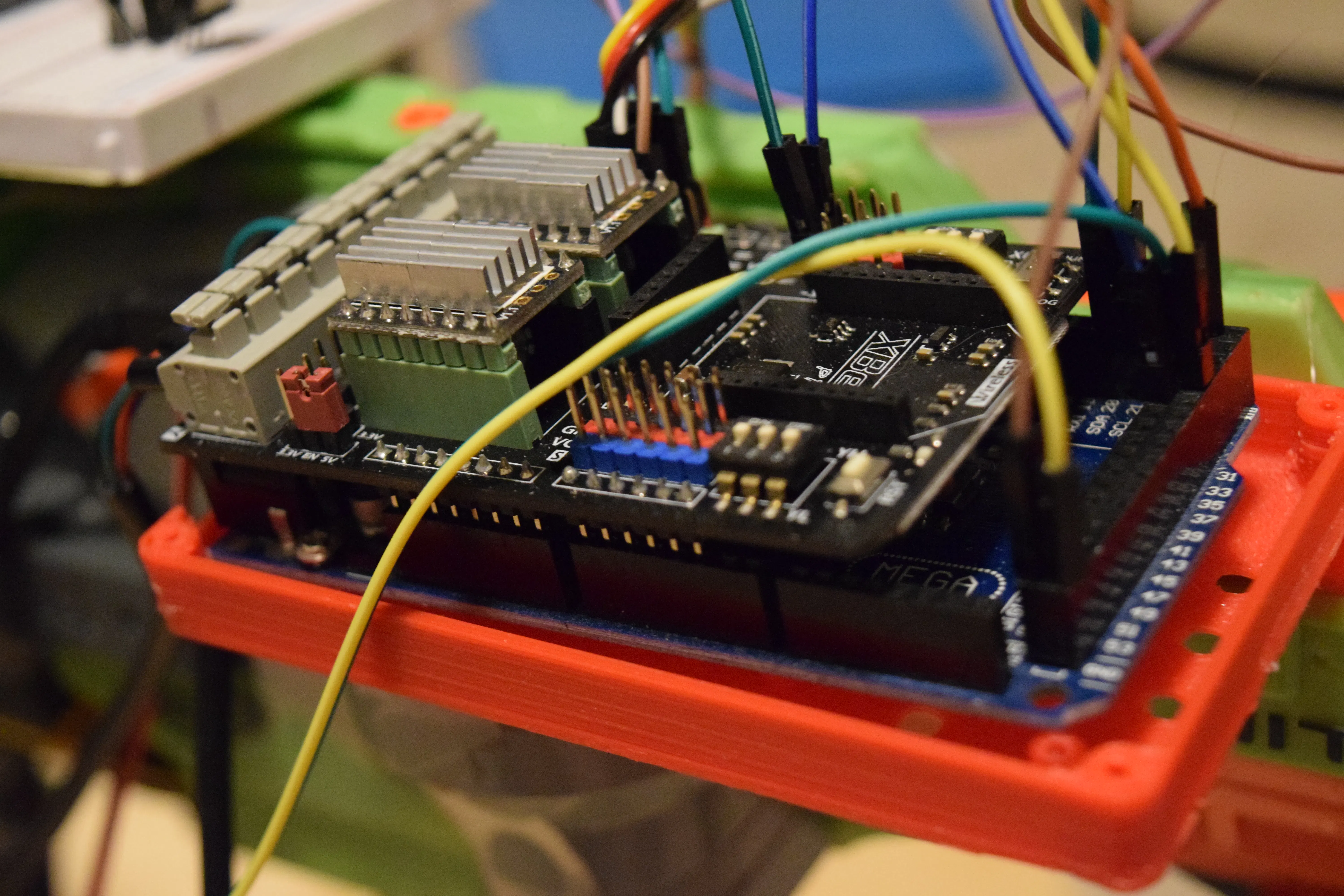## Code

### Schematic

C/C++
```#include <math.h>
#include <Arduino.h>
#include "BasicStepperDriver.h"
#include <Servo.h>
#include <Pixy2I2C.h>

//X is pitch, Y is yaw

const int pins[] = {6,7,8,5,4,12}; //MX STEP, DIR, EN, MY STEP, DIR, EN
const int limit_switch = 26, laser_pin = 11, spool_pin = 10, servo_pin = 13, distance_trig = 29, distance_echo = 30;
double velocity = 21.336;
double velocity_squared = 455.225;
float current_angle = 0.0;
float hyp_distance; //distance from gun to target in meters

#define X_MID 164
#define Y_MID 150

#define G 9.8
#define STP_PER_DEG_YAW 3.333
#define STP_PER_DEG_PITCH 184859

#define MICROSTEPS 32
#define RPM 120

#define MOTOR_STEPS_Y 200
#define MOTOR_STEPS_X 1036

//17.7777 steps / degree

BasicStepperDriver pitch_stepper(MOTOR_STEPS_X, pins, pins);
BasicStepperDriver yaw_stepper(MOTOR_STEPS_X, pins, pins);
Servo trigger;
Pixy2I2C pixy;

enum States {
ACQUIRE,
POSITION,
SPOOL,
FIRE,
WIND_DOWN,
RETURN
};

States state = ACQUIRE;

void setup() {
Serial.begin(115200);
init_pins();
delay(1000);
//home_pitch();
pixy.init();
}

void loop() {
switch(state){
case ACQUIRE:
acquire_target();
state = POSITION;
digitalWrite(laser_pin,HIGH);
break;
case POSITION:
Serial.println("positioning");
position_gun();
state = SPOOL;
break;
case SPOOL:
Serial.println("spooling");
digitalWrite(spool_pin,HIGH);
delay(5000);
state = FIRE;
break;
case FIRE:
fire_gun();
state = WIND_DOWN;
break;
case WIND_DOWN:
Serial.println("winding down");
digitalWrite(spool_pin,LOW);
delay(2000);
state = RETURN;
digitalWrite(laser_pin,LOW);
state = ACQUIRE;
break;
}
}

void fire_gun(){
Serial.println("Firing gun!");
trigger.write(108);
delay(400);
trigger.write(90);
delay(2000);
}

void position_gun(){
float x, y;
hyp_distance = ping();
hyp_distance /= 100;
while(!hyp_distance){
hyp_distance = ping();
hyp_distance /= 100;
}Serial.println(hyp_distance);
x = cos(current_angle) * hyp_distance;
y = sin(current_angle) * hyp_distance;
float target_angle = get_angle(x,y);
target_angle /= 100;
Serial.println(target_angle);
move_pitch(target_angle - current_angle);
current_angle = target_angle;
}

void acquire_target(){
int x=0, y=0;
long steps_taken=0;
bool lock = false;
while(!lock){
pixy.ccc.getBlocks();
if(pixy.ccc.numBlocks){
x = pixy.ccc.blocks.m_x;
y = pixy.ccc.blocks.m_y;
Serial.print("Target seen at location X: ");Serial.print(x);Serial.print(", Y: ");Serial.println(y);
if(x <= (X_MID - DEADZONE)){ //If too far left, move gun left
move_yaw(1);
}
else if(x >= (X_MID + DEADZONE)){
move_yaw(-1);
}
else if(y <= (Y_MID - DEADZONE)){ //too far up, move gun up
pitch_stepper.move(33152);
steps_taken += 33152;
}
else if(y >= (Y_MID + DEADZONE)){
pitch_stepper.move(33152);
steps_taken += 33152;
}
else{
lock = true;
Serial.print("Target locked at location X: ");Serial.print(x);Serial.print(", Y: ");Serial.println(y);
Serial.print("Steps taken: ");Serial.println(steps_taken);
}
}

}
current_angle = steps_taken / STP_PER_DEG_PITCH;
Serial.print("Current angle: ");Serial.println(current_angle);
}

void init_pins(){
pinMode(pins,OUTPUT);
pinMode(pins,OUTPUT);
pinMode(limit_switch, INPUT_PULLUP);
pinMode(laser_pin, OUTPUT);
pinMode(spool_pin, OUTPUT);
pinMode(distance_echo, INPUT);
pinMode(distance_trig, OUTPUT);
digitalWrite(pins,LOW);
digitalWrite(pins,LOW);
digitalWrite(laser_pin,LOW);
digitalWrite(spool_pin,LOW);
trigger.attach(servo_pin);
pitch_stepper.begin(RPM, MICROSTEPS);
yaw_stepper.begin(5, MICROSTEPS);
trigger.write(90);
}

void move_yaw(float degrees){
yaw_stepper.move(degrees*STP_PER_DEG_YAW*32);
}

void move_pitch(float degrees){
current_angle += degrees;
pitch_stepper.move(degrees*STP_PER_DEG_PITCH);
}

float get_angle(float distance, float height){
float i = 2 * height * 455.225;
float j = G * distance * distance;
i += j;
j = 9.8 * i;
i = sqrt(pow(velocity_squared,2) - j);

return atan((velocity_squared-i) / (G * distance))*(180/PI);
}

float ping(){
Serial.println("Getting distance...");
long duration;
digitalWrite(distance_trig, LOW);
delayMicroseconds(5);
digitalWrite(distance_trig, HIGH);
delayMicroseconds(10);
digitalWrite(distance_trig, LOW);
duration = pulseIn(distance_echo, HIGH);
return duration / 2 / 29.1; //distance in meters
}

void home_pitch(){
pitch_stepper.rotate(720);
}
pitch_stepper.move(-32);
}
pitch_stepper.rotate(2880*2);
}
```

## Credits

### Arduino “having11” Guy

81 projects • 539 followers
18 year-old IoT and embedded systems enthusiast. Also an intern at Hackster.io who loves working on projects and sharing knowledge.